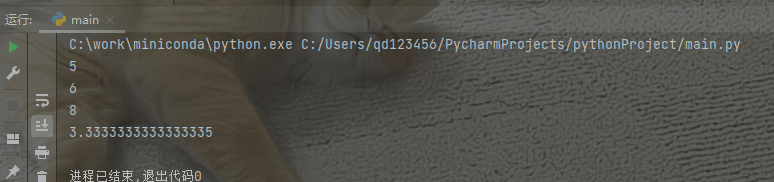# 22個Python的萬用公式分享

## 1、一次性進行多個數值的輸入

```# 確定數值的輸入時

num1,num2 = map(int,input().split())
print("num1:",num1)
print("num2:",num2)

# 不確定數值的輸入時

list1 = list(map(int,input().split()))
print("list1:",list1)

```## 2、同時獲取索引和數值

```list2 = [1,2,3,4,5,6]
for k, v in enumerate(list2):
if k % 2 == 0:
print("v**2:",v**2)
else:
print("v:",v)
```## 3、對象內存占用量

```from sys import getsizeof
num = 1
print(getsizeof(num))
```## 4、對象內存地址的查詢

```num1 = 20
str1 = "hello world"
print(id(num1))
print(id(str1))
```## 5、檢查列表、字符串是否有相同的元素

```def CheckStr(gen1,gen2):
return sorted(gen1) == sorted(gen2)

print(CheckStr("python","python"))
print(CheckStr([1,2,3],[3,2,1]))
```## 6、合並字典

```dict1 = {"name":['Jame','Alice'],"num":["212019","312016"]}
dict2 = {"sex":["M","F"]}

# 方法1

finaldict = {**dict1,**dict2}
print(finaldict)

# 方法2

finaldict = dict1.copy()
finaldict.update(dict2)
print(finaldict)```## 7、檢查文件是否存在

```import os

def CheckFile():
print("文件夾存在:",os.path.exists("data"))
if not os.path.exists("data"):
os.mkdir("data")
CheckFile()
```## 8、對列表元素進行操作

```list8 = range(1,8)
list_squares = [i**2 for i in list8]
print(list_squares)
```## 9、將兩個列表轉換為字典

```list1 = ['James','Alice','Hoton']
list2 = [88,86,91]

# 方法1 利用zip內置函數
dict1 = dict(zip(list1,list2))

# 方法2 去除dict的隱式轉換
dict2 = {key:value for key,value in zip(list1,list2)}

# 方法3 利用for循環
dict3 = {}
for k, v in zip(list1,list2):
if k not in dict3.keys():
dict3[k] = v

print("dict1:",dict1)
print("dict2:",dict2)
print("dict3:",dict3)
```## 10、字符串列表的排序

```list1 = ['James','Alice','Hoton','Cris']
print(sorted(list1,key=lambda x:x.lower()))  # 按照字符串的第一個字母排序
print(sorted(list1,key=lambda x:x.lower()[-1])) # 按照字符串的最後一個字母排序
```## 11、利用if和else對列表進行處理

```list11 = list(range(1,20))
print("偶數平方:",[i**2 if i % 2 == 0 else i for i in list11])
```## 12、合並兩個列表

```list1 = ["1","2","3","4"]
list2 = ["one","two","three","four"]

new_list = [x + y for x,y in zip(list1,list2)]
print("逐元素相加：",new_list)
```## 13、對字典列表進行排序

```dict1 = [
{"name":"James",
"num":25},
{"name":"Alice",
"num":39},
{"name":"Hoton",
"num":35}
]

# 方法1 利用字典的sort函數
dict1.sort(key=lambda item:item["num"])
print(dict1)

# 方法2 利用sorted函數
dict1 = sorted(dict1,key=lambda item:item["num"])
print(dict1)
```## 14、計算程序執行的時間

```from time import sleep
def funcl():
for i in range(10000000):
a = i
sleep(2)

# 方法1
from datetime import datetime
start = datetime.now()
funcl()
print("程序執行所用的時間為：",datetime.now()-start)

# 方法2
import time

start_time = time.time()
funcl()
print("程序執行所用的時間為：",time.time()-start_time)
```## 15、檢查是否包含子字符串

```str_list = ["小圓愛python","hello world","小圓愛python","kaka小圓"]
keywords = 'python'

for strs in str_list:
if keywords in strs:
print(strs)
```## 16、格式化字符串

```name = "愛坤"
num = 100

# 方法1 字符串相加
print("我的名字是"+name+",我的成績是"+str(num)+"。")

# 方法2 Python3 中的F-strings
print(f"我的名字是{name},我的成績是{num}。")

# 方法3 join函數
print(''.join(["我的名字是",name,"，我的成績是",str(num),"。"]))

# 方法4 操作字符處理
print("我的名字是%s，我的成績是%d。" % (name,num))

# 方法5 format(python2.7以上的版本)
print("我的名字是{},我的成績是{}。".format(name,num))
```## 17、錯誤捕捉

```# 錯誤1 扣除為0
try:
num1 = 10
num2 = 0
print(num1 / num2)
except ZeroDivisionError :
print("除數不能為0")

print("=================")

# 錯誤2 找不到文件
try:
with open("data.txt",'r') as fr:
except IOError:
print("該文件不存在")
finally:
print("程序執行結束")```## 18、列表元素頻率統計

```list1 = [1,2,3,4,5,6,7,8,3,3,4,5,2,3,2]

# 方法1 利用for循環統計
frequ_dict = {}
for i in list1:
if i in frequ_dict.keys():
frequ_dict[i] += 1
else:
frequ_dict[i] = 1
print(frequ_dict)

# 方法2 李彤Counter類
from collections import Counter
Counter = Counter(list1)
print(Counter.most_common())
```## 19、簡易計算器制作

```from operator import add, sub, truediv, mul

operation = {
"-" : sub,
"/" : truediv,
"*" : mul,
"**" : pow
}

print(operation['+'](2,3))
print(operation['*'](2,3))
print(operation['**'](2,3))
print(operation['/'](10,3))
```## 20、鏈式函數調用

```def add(x,y):
return x + y

def sub(x,y):
return x - y

x,y = 2,3
print((sub if x > y else add)(x,y))
# 通過條件判斷執行的函數
```## 21、兩個數值交換

Python中的交換，不僅僅可以直接通過a,b = b,a的方式進行數值的交換，而且還可以進行列表等可迭代對象的交換。

```x, y = 2, 3
print("交換前：x={}, y={}".format(x, y))
x, y = y, x
print("交換後：x={}, y={}".format(x, y))

x, y = [2, 3], [4,5]
print("交換前: x={}, y={}".format(x, y))
x, y = y, x
print("交換後：x={}, y={}".format(x, y))
```## 22、檢查是否有重復元素

```list1 = [1,2,3,4,2,4,5]
list2 = [1,2,3,4,5,6,7]
print("list1有重復元素：",len(list1) != len(set(list1)))
print("list2有重復元素：",len(list2) != len(set(list2)))
```